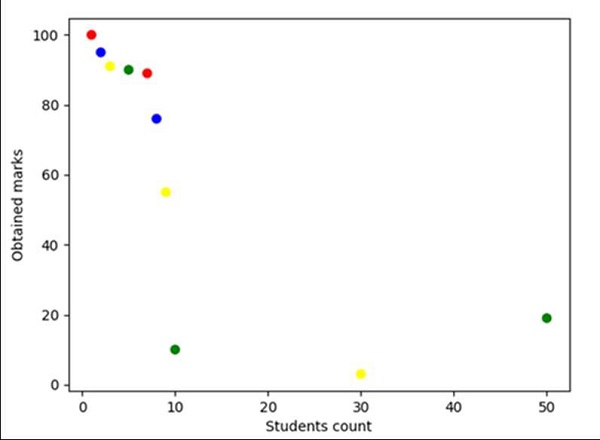# How to make several plots on a single page using matplotlib in Python?

Using Pandas, we can create a data frame and create a figure and axis. After that, we can use the scatter method to draw points.

## Steps

• Create lists of students, marks obtained by them, and color codings for each score.

• Make a data frame using Panda’s DataFrame, with step 1 data.

• Create fig and ax variables using subplots method, where default nrows and ncols are 1.

• Set the X-axis label using plt.xlabel() method.

• Set the Y-axis label using plt.ylabel() method.

• A scatter plot of *y* vs. *x* with varying marker size and/or color.

• To show the figure, use plt.show() method.

## Example

from matplotlib import pyplot as plt
import pandas as pd

no_of_students = [1, 2, 3, 5, 7, 8, 9, 10, 30, 50]
marks_obtained_by_student = [100, 95, 91, 90, 89, 76, 55, 10, 3, 19]
color_coding = ['red', 'blue', 'yellow', 'green', 'red', 'blue', 'yellow', 'green', 'yellow', 'green']

df = pd.DataFrame(dict(students_count=no_of_students,
marks=marks_obtained_by_student, color=color_coding))

fig, ax = plt.subplots()

plt.xlabel('Students count')
plt.ylabel('Obtained marks')

ax.scatter(df['students_count'], df['marks'], c=df['color'])

plt.show()

## Output